+

Author: Sophia Tutorial
##### Description:

(more)

Sophia’s self-paced online courses are a great way to save time and money as you earn credits eligible for transfer to many different colleges and universities.*

No credit card required

46 Sophia partners guarantee credit transfer.

299 Institutions have accepted or given pre-approval for credit transfer.

* The American Council on Education's College Credit Recommendation Service (ACE Credit®) has evaluated and recommended college credit for 33 of Sophia’s online courses. Many different colleges and universities consider ACE CREDIT recommendations in determining the applicability to their course and degree programs.

Tutorial
what's covered
1. Square Roots
2. Cube Roots
3. Higher Roots

# 1. Square Roots

Square roots are the most common type of radical used. A square root “unsquares” a number. For example, because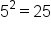we say the square root of 25 is 5. The square root of 25 is written as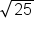.

did you know
The radical sign, when first used was an R with a line through the tail, similar to our prescription symbol today. The R came from the latin, “radix”, which can be translated as “source” or “foundation”. It wasn’t until the 1500s that our current symbol was first used in Germany (but even then it was just a check mark with no bar over the numbers!)

The following example gives several square roots: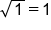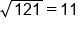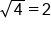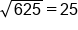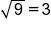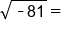Undefined

hint
In the last example,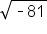is undefined, as negatives have no square root. This is because if we square a positive or a negative, the answer will be positive (or zero). Thus we can only take square roots of non-negative numbers. In another lesson, we define a method we can use to work with and evaluate negative square roots, but for now we will simply say they are undefined.

Not all numbers have a nice even square root. For example, if we found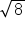on our calculator, the answer would be 2.828427124746190097603377448419... and even this number is a rounded approximation of the square root. Decimal approximations will work in most cases, but you may need the exact value, in which case you will express using the radical symbol, rather than expressing as a decimal.

hint
When evaluating square roots, look for perfect squares. Perfect square are numbers such as 2, 4, 9, 16 (they are integers squared). You can also use your calculator's radical button,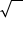, or apply a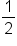exponent.

# 2. Cube Roots

Just like square roots undo squaring a number, cube roots undo cubing a number. For example, because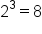, we say that the cube root of 8 is 2. The cube root of 8 is written as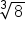with a 3 as the index of the radical, to indicate a cube root.

Here are some examples of cube roots:Notice that the cube root of a negative number is results in a real number. This is because a negative number cubed is a negative number. So while square roots of negative numbers are non-real numbers, cube roots of negative numbers are real numbers. This actually holds true for all even roots and odd roots.

big idea
Taking an even root of a negative number leads to a non-real solution, because any negative number raised to an even power is positive. However, taking an odd root of a negative number leads to a real number solution, because raising a negative number to an odd power results in a negative number.
hint
When evaluating a cube root, look for perfect cubes. These will result in an integer, because perfect cubes are integers cubed. Some examples of perfect cubes are: 1, 8, 27, 64, and 125. You can also use your calculator's cube root button,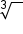, or raise to the fractional power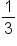.

# 3. Higher Roots

While square and cube roots are the most common type of radical we work with, we can take higher roots of numbers as well: cube roots, fourth roots, fifth roots, etc. Consider this definition of radicals:

formula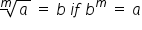The small letter m inside the radical is called the index. It tells us which root we are taking, or which power we are “un-doing”. For square roots the index is 2. As this is the most common root, the two is not usually written.

did you know
The word for root comes from the French mathematician Franciscus Vieta in the late 16th century.

The following example includes several higher roots.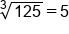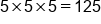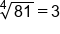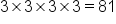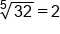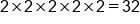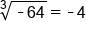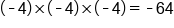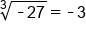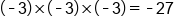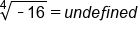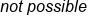This last example is not possible, or undefined, because if we take any positive or negative number to the fourth power, the answer will be positive (or zero).or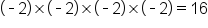. Thus we can only take the fourth roots of non-negative numbers.

hint
We must be careful of a few things as we work with higher roots. First, its important not to forget to check the index on the root.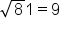but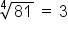This is because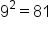and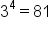. Another thing to watch out for is negative value under roots. We can take an odd root of a negative number, because a negative number raised to an odd power is still negative. However, we cannot take an even root of a negative number, because a negative number raised to an even power is positive.

summary
If you use a calculator to evaluate the radical, you first type in the radical button and then you type in the number. If your calculator does not have the necessary nth root button, you're going to use a fractional exponent and type it in as caret, open parentheses, one divided by n, close parentheses. And again, your n number is just the index of your radical. And finally, the nth root of a negative number will not evaluate to be a real number if n is even-- like square root-- but it will evaluate to be a real number if n is odd-- like the cubed root.

Source: Adapted from "Beginning and Intermediate Algebra" by Tyler Wallace, an open source textbook available at: http://wallace.ccfaculty.org/book/book.html

Rating